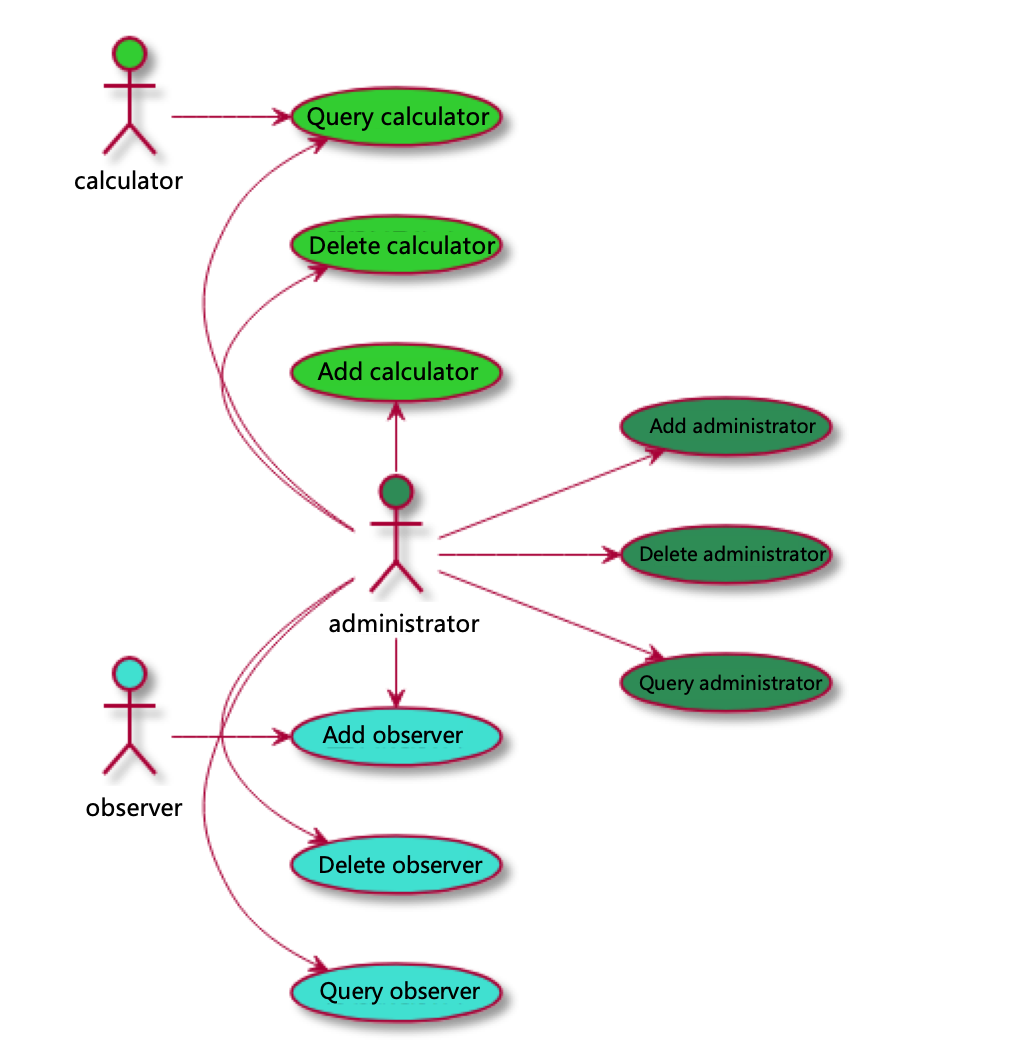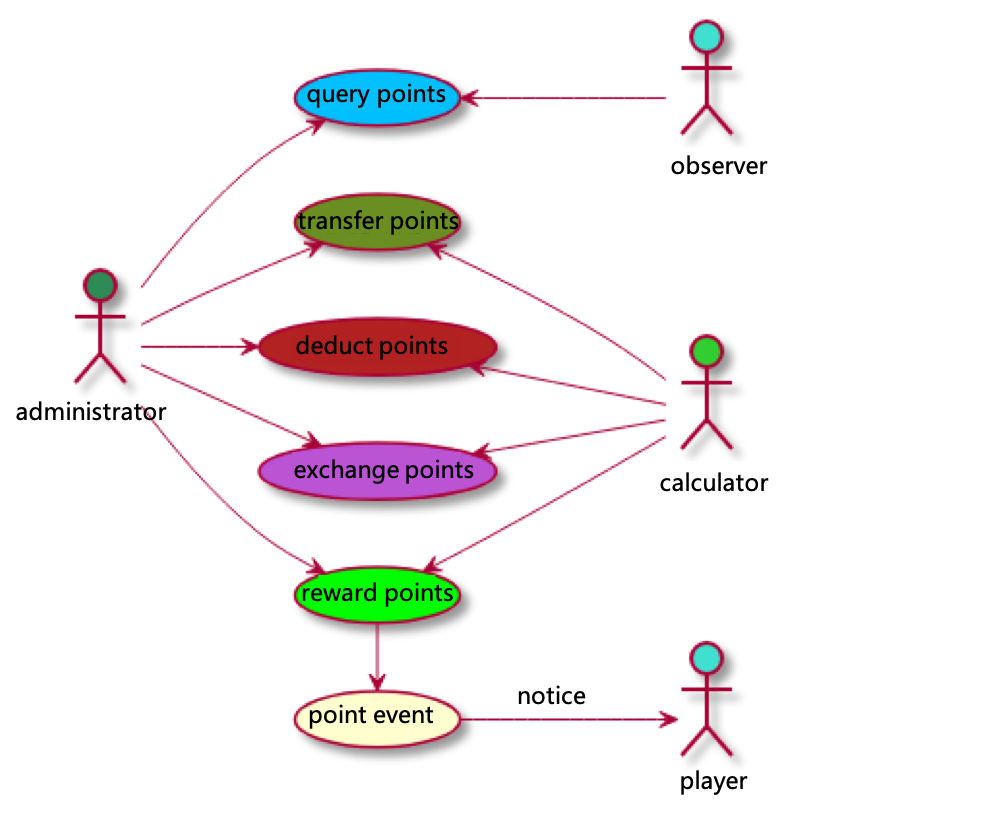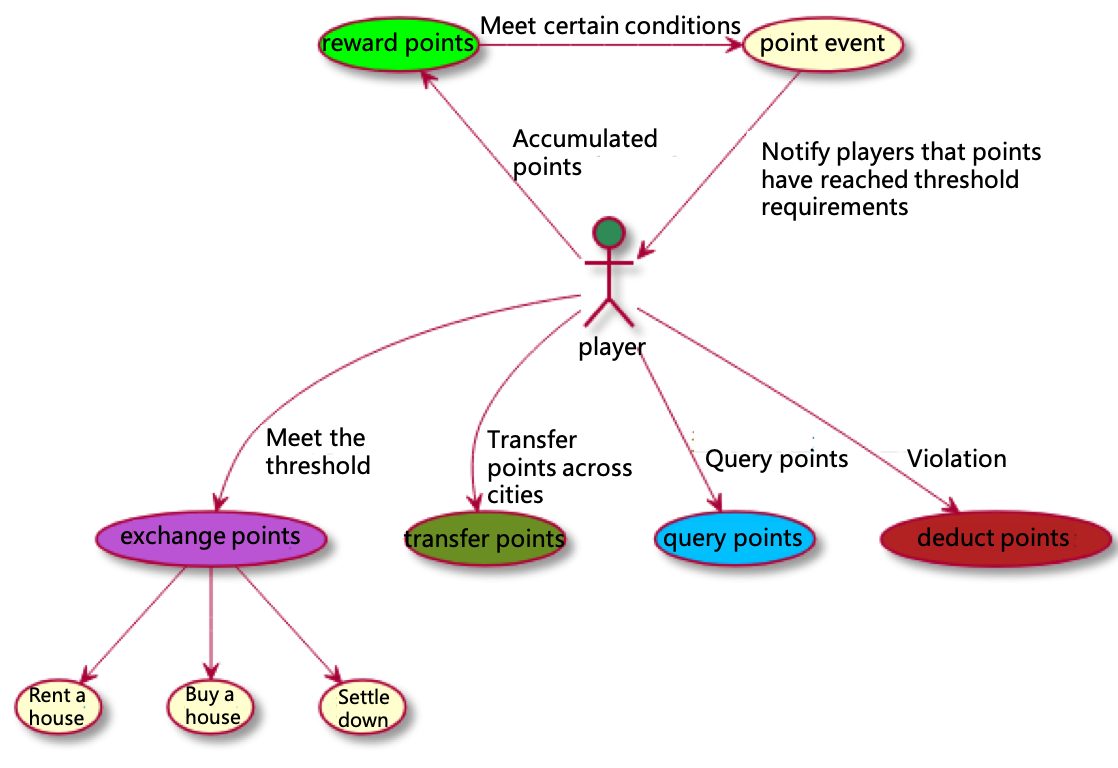Search
Document Center

# Contract Examples

Last Updated: Jan 09, 2020

This topic is a reference to help you design a contract as required. The smart contract-based system for managing housing rental points is used as an example.

Note: The following example is for reference only and cannot be used in production environments.

## Background

The blockchain smart contract-based system used to manage housing rental points efficiently provides services for individuals and organizations. It helps those involved in city development find affordable houses to rent and those who contribute to long-term city development to find houses to buy. With the blockchain, big data, and other cutting-edge technologies, this system provides a housing rental point mechanism based on the main characteristics of the housing rental market, incentives, and rental behavior. This system implements an effective, scientific system to manage the lifecycles of housing rental points and creates a dynamic, healthy, organized, and sustainable housing rental ecosystem.

## Purpose

The system uses the blockchain platform to protect privacy and prevent data from being tampered with. This system provides a point decision-making engine and a point evaluation mechanism and gives a comprehensive point solution for housing rental. This implements fairness, impartiality, and transparency. This solution is horizontally expandable. It relies on its unchanged fundamental technologies and data. This system supports the construction of diverse point systems (such as an economic credit point system or green living point system) for the future and can be expanded to an urban credit system for medical education, financial lending, green living, and other scenarios.

## Contract design

### Roles

In the system used to manage housing rental points, there are four roles: administrators, calculators, observers, and players. Administrators, calculators, and observers conduct operations on smart contracts, and each role can correspond to multiple persons. Players are the core users of smart contracts for rental points.

#### Permissions on roles

• A calculator can view information about calculators.
• An observer can view information about observers.#### Permissions on points

• An administrator can reward, query, transfer, deduct, and exchange points.
• A calculator is specified by the administrator, and can be a person or system service. A calculator can reward, transfer, deduct, and exchange points.
• An observer is specified by the administrator, and can be a person or system service. An observer can only query points.
• A player is a user of the housing rental point system and benefits from this system.
• A player can obtain points by using observer permissions to query rental points from the external service system proxy of an organization.
• The external service system for rental points rewards or deducts points for a player based on player’s performance.
• A player can transfer or exchange points by using administrator permissions to query rental points from the external service system proxy of an organization.### Point management

Smart contracts for rental points provide the following service methods: awardScore, queryScore, exchangeScore, deductScore, transferScore, and SCORE_EQUITY_NOTICE triggered after points reach the upper limit.#### Point reward (awardScore)

Accumulates points for players while they rent houses based on the rental period and behavior.

#### Point query (queryScore)

Enables players to obtain their points by using the observer permissions of the external service system for rental points.

#### Point exchange (exchangeScore)

Allows players to receive benefits, such as preferential rental, qualifications for buying a house, and qualifications for settling down, after their points reach a specific threshold.

#### Point deduction (deductScore)

Deducts points for player violations while they rent houses. Housing rental points are used to encourage players. In regards to information falsification, player points are deducted, players are disqualified, and their rights and interests to exchange points are reclaimed based on the severity of the circumstances.

#### Point event (SCORE_EQUITY_NOTICE)

When point rights and interests are added in real time, they will be checked. If points reach a specific threshold, an event for notifying related rights and interests will be triggered. The external service system notifies players of these rights and interests.

#### Point transfer (transferScore)

If a player moves to another city, his/her points can be transferred to the point system in the destination city. To call the original contract, the target contract must have the `awardScore` method, and provide operation permissions for the original contract. Make sure that the address of the original contract must be the administrator or calculator.

## Smart contract code

```
`pragma solidity ^0.4.20;`

`contract LeasingScoreManager {`
`    identity[] adminList;`
`    identity[] observerList;`
`    identity[] calculatorList;`

`    //Record elements that are set in management arrays.`
`    uint adminDeled;`
`    uint observerDeled;`
`    uint calculatorDeled;`

`    mapping (bytes32 => uint) leasingScore;`

`    enum ScoreAction {`
`        //Perform point reward based on behavior.`
`        ActionAwardScore,`
`        //Exchange specified points.`
`        ActionExchangeScore,`
`        //Deduct points due to violations.`
`        ActionDeductScore,`
`        //Query points.`
`        ActionQueryScore`
`        //Transfer points to another contract.`
`    }`

`    enum UserRole {`
`        RoleAdmin, //Administrator`
`        RoleCalculator, //Calculator`
`        RoleObserver, //Observer`
`        RolePlayer //Player`
`    }`

`    //Whether the calculator is valid.`
`    event VALID(bool valid, UserRole role);`
`    //Whether the administrator, observer, or calculator exists.`
`    event USER_EXIST(bool exist, UserRole role);`
`    //Point event`
`    event SCORE_OPERATOR(ScoreAction action, string describe);`
`    //Incorrect operation on points`
`    event SCORE_ERROR(ScoreAction action, string describe);`
`    //Notification of rights and interests`
`    event SCORE_EQUITY_NOTICE(string action, uint score, string describe);`

`    constructor() public {`
`        adminList.push(msg.sender);`
`        adminList.push(this);`
`    }`

`    function indexAdmin(identity admin) view returns (uint) {`
`        for (uint i = 0; i < adminList.length; i++) {`
`            if (adminList[i] == admin) {`
`                return i;`
`            }`
`        }`
`        return adminList.length;`
`    }`

`    function validAdmin(identity admin) view returns (bool) {`
`        return indexAdmin(admin) < adminList.length;`
`    }`

`    function indexCalculator(identity calculator) view returns (uint) {`
`        for (uint i = 0; i < calculatorList.length; i++) {`
`            if (calculatorList[i] == calculator) {`
`                return i;`
`            }`
`        }`
`        return calculatorList.length;`
`    }`

`    function validCalculator(identity calculator) view returns (bool) {`
`        return indexCalculator(calculator) < calculatorList.length;`
`    }`

`    function indexObserver(identity observer) view returns (uint) {`
`        for (uint i = 0; i < observerList.length; i++) {`
`            if (observerList[i] == observer) {`
`                return i;`
`            }`
`        }`
`        return observerList.length;`
`    }`

`    function validObserver(identity observer) view returns (bool) {`
`        return indexObserver(observer) < observerList.length;`
`    }`

`    modifier onlyAdmin {`
`        bool isValid = validAdmin(msg.sender);`
`        emit VALID(isValid, UserRole.RoleAdmin);`
`        require(isValid);`
`        _;`
`    }`

`    modifier onlyCalculatorOrAdmin {`
`        bool isValid = validAdmin(msg.sender);`
`        if(isValid) {`
`            emit VALID(isValid, UserRole.RoleAdmin);`
`        } else {`
`            isValid = validCalculator(msg.sender);`
`            emit VALID(isValid, UserRole.RoleCalculator);`
`        }`
`        require(isValid);`
`        _;`
`    }`

`    modifier onlyObserverOrAdmin {`
`        bool isValid = validAdmin(msg.sender);`
`        if(isValid) {`
`            emit VALID(isValid, UserRole.RoleAdmin);`
`        } else {`
`            isValid = validObserver(msg.sender);`
`            emit VALID(isValid, UserRole.RoleObserver);`
`        }`
`        require(isValid);`
`        _;`
`    }`

`    function addAdmin(identity admin) public onlyAdmin {`
`        bool isExist = validAdmin(admin);`
`        emit USER_EXIST(isExist, UserRole.RoleAdmin);`
`        require(!isExist);`
`        if(adminDeled > 0) {`
`            uint deled = 1;`
`            for (uint i = 0; i < adminList.length; i++) {`
`                if(deled&adminDeled != 0) {`
`                    adminList[i] = admin;`
`                    adminDeled ^= deled;`
`                    break;`
`                }`
`                deled <<= 1;`
`            }`
`        } else {`
`            adminList.push(admin);`
`        }`
`    }`

`    function removeAdmin(identity admin) public onlyAdmin {`
`        uint index = indexAdmin(admin);`
`        bool isValid = index != adminList.length;`
`        emit USER_EXIST(isValid, UserRole.RoleAdmin);`
`        require(isValid);`
`        delete adminList[index];`
`        adminDeled ^= 1 << index;`
`    }`

`    function queryAdmins() view public onlyAdmin returns (identity[]) {`
`        return adminList;`
`    }`

`    function addCalculator(identity calculator) public onlyAdmin {`
`        bool isExist = validCalculator(calculator);`
`        emit USER_EXIST(isExist, UserRole.RoleCalculator);`
`        require(!isExist);`
`        if(calculatorDeled > 0) {`
`            uint deled = 1;`
`            for (uint i = 0; i < calculatorList.length; i++) {`
`                if(deled&calculatorDeled != 0) {`
`                    calculatorList[i] = calculator;`
`                    calculatorDeled ^= deled;`
`                    break;`
`                }`
`                deled <<= 1;`
`            }`
`        } else {`
`            calculatorList.push(calculator);`
`        }`
`    }`

`    function removeCalculator(identity calculator) public onlyAdmin {`
`        uint index = indexCalculator(calculator);`
`        bool isValid = index < calculatorList.length;`
`        emit USER_EXIST(isValid, UserRole.RoleCalculator);`
`        require(isValid);`
`        delete calculatorList[index];`
`        calculatorDeled ^= 1 << index;`
`    }`

`    function queryCalculators() view public onlyCalculatorOrAdmin returns (identity[]) {`
`        return calculatorList;`
`    }`

`    function addObserver(identity observer) public onlyAdmin {`
`        bool isExist = validObserver(observer);`
`        emit USER_EXIST(isExist, UserRole.RoleObserver);`
`        require(!isExist);`
`        if(observerDeled > 0) {`
`            uint deled = 1;`
`            for (uint i = 0; i < observerList.length; i++) {`
`                if(deled&observerDeled != 0) {`
`                    observerList[i] = observer;`
`                    observerDeled ^= deled;`
`                    break;`
`                }`
`                deled <<= 1;`
`            }`
`        } else {`
`            observerList.push(observer);`
`        }`
`    }`

`    function removeObserver(identity observer) public onlyAdmin {`
`        uint index = indexCalculator(observer);`
`        bool isValid = index < observerList.length;`
`        emit USER_EXIST(isValid, UserRole.RoleObserver);`
`        require(isValid);`
`        delete observerList[index];`
`        observerDeled ^= 1 << index;`
`    }`

`    function queryObservers() view public onlyObserverOrAdmin returns (identity[]) {`
`        return observerList;`
`    }`

`    function checkScoreEquity(uint balance, uint score) {`
`        uint total = balance + score;`
`        if(total >= 100 && balance < 100) {`
`            emit SCORE_EQUITY_NOTICE("RentConcessions", total, "Citizens enjoy a 90% discount on rental housing");`
`        }`
`        if(total >= 200 && balance < 200) {`
`            emit SCORE_EQUITY_NOTICE("RentConcessions_1", total, "Citizens enjoy a 80% discount on rental housing");`
`        }`
`        if(total >= 300 && balance < 300) {`
`            emit SCORE_EQUITY_NOTICE("PurchaseDiscount", total, "Citizens enjoy a 90% discount on purchase housing");`
`        }`
`    }`

`    //Point reward`
`    function awardScore(bytes32 player, uint score, string describe) public onlyCalculatorOrAdmin {`
`        uint balance = leasingScore[player];`
`        leasingScore[player] = balance + score;`
`        emit SCORE_OPERATOR(ScoreAction.ActionAwardScore, describe);`
`        checkScoreEquity(balance, score);`
`    }`

`    //Point exchange by the player. If points are not enough, points will not be deducted, and a failure event will be reported.`
`    function exchangeScore(bytes32 player, uint score, string describe) public onlyCalculatorOrAdmin returns (bool) {`
`        emit SCORE_OPERATOR(ScoreAction.ActionExchangeScore, describe);`
`        if(leasingScore[player] >= score) {`
`            leasingScore[player] -= score;`
`            return true;`
`        }`
`        emit SCORE_ERROR(ScoreAction.ActionExchangeScore, "Score not enough to exchange");`
`        return false;`
`    }`

`    //Point deduction that is not initiated by the player. If points are not enough for the deduction, points will be cleared, and a failure event will be reported.`
`    function deductScore(bytes32 player, uint score, string describe) public onlyCalculatorOrAdmin {`
`        emit SCORE_OPERATOR(ScoreAction.ActionDeductScore, describe);`
`        uint balance = leasingScore[player];`
`        if(balance >= score) {`
`            leasingScore[player] -= score;`
`        } else {`
`            if(balance != 0) {`
`                leasingScore[player] = 0;`
`            }`
`            emit SCORE_ERROR(ScoreAction.ActionDeductScore, "Score not enough to deduct");`
`        }`
`    }`

`    //Point query`
`    function queryScore(bytes32 player, string describe) view public onlyObserverOrAdmin returns (uint) {`
`        emit SCORE_OPERATOR(ScoreAction.ActionQueryScore, describe);`
`        return leasingScore[player];`
`    }`

`    //String combination`
`    function stringAdd(string a, string b) returns(string){`
`        bytes memory _a = bytes(a);`
`        bytes memory _b = bytes(b);`
`        bytes memory res = new bytes(_a.length + _b.length);`
`        for(uint i = 0;i < _a.length;i++)`
`            res[i] = _a[i];`
`        for(uint j = 0;j < _b.length;j++)`
`            res[_a.length+j] = _b[j];  `
`        return string(res);`
`    }`

`    //Point transfer to another contract`
`    function transferScore(bytes32 player, uint score, LeasingScoreManager toContract, string describe) public onlyCalculatorOrAdmin {`
`        //Point deduction if points are enough`
`        describe =  stringAdd("Transfer score: ", describe);`
`        require(exchangeScore(player, score, describe));`
`        toContract.awardScore(player, score, describe);`
`    }`
`}`
```